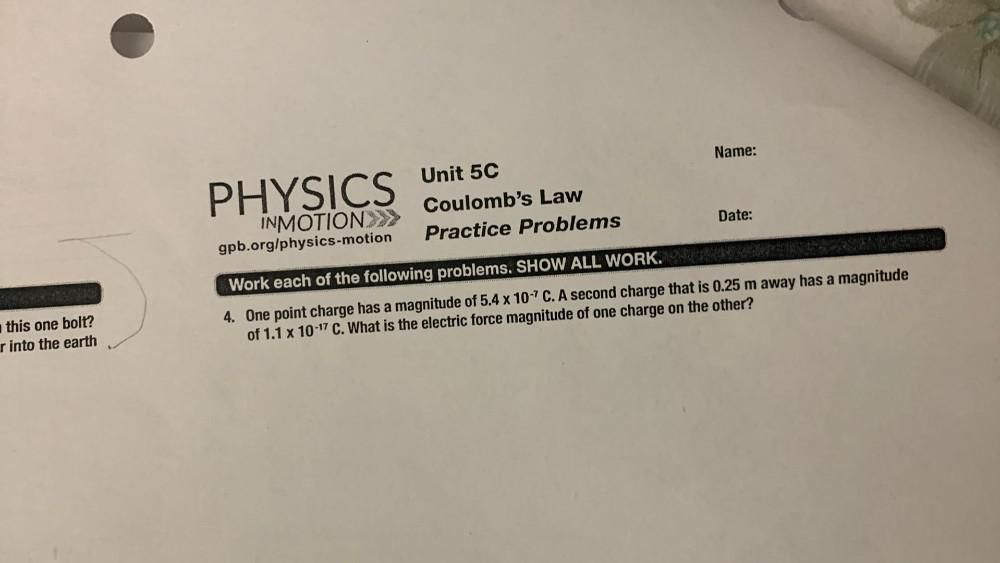Question:

# Name: PHYSICS Unit 5C Coulomb's Law Practice Problems Date: INMOTION>>> gpb.org/physics-motion Work each of the following probleName: PHYSICS Unit 5C Coulomb's Law Practice Problems Date: INMOTION>>> gpb.org/physics-motion Work each of the following problems. SHOW ALL WORK. 4. One point charge has a magnitude of 5.4 x 10-7C. A second charge that is 0.25 m away has a magnitude of 1.1 x 10-17 C. What is the electric force magnitude of one charge on the other? this one bolt? r into the earth